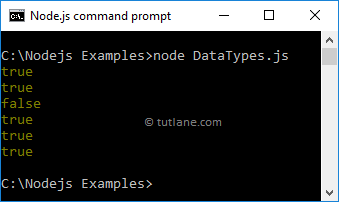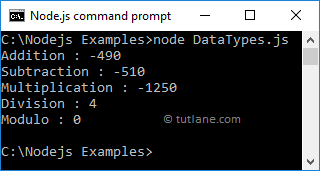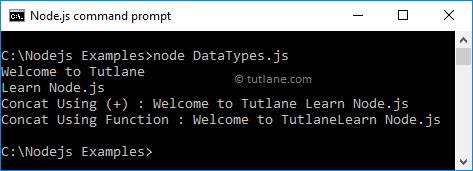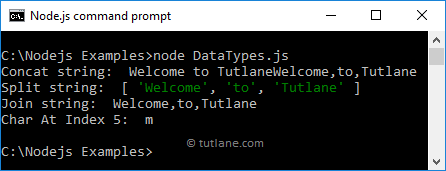# Node.js Basics

As we discussed in the introduction to node.js, Node.js is a purely open-source JavaScript runtime environment and it built on Google Chrome’s V8 JavaScript Engine so the node.js is faster in operation and also provides an effective I/O operation.

The node.js will support all the JavaScript functionalities so the syntax of JavaScript in node.js is same as the browser’s JavaScript syntax.

## Dynamic Typing

The node.js will support dynamic or loose typing same as JavaScript so any variable can be assigned or re-assigned values of all the types.

In node.js, we can use `var` keyword to declare a variable of any type like as shown below.

var x = 20; // x is now number

x = 'welcome'; // x is now string

x = true; // x is now boolean

## Objects & Functions

In JavaScript, object is a mapping between the keys and values. In object, keys are strings and values can be anything. In node.js, the object declaration will be same like browser’s JavaScript.

Following is the example of defining an object in node.js.

var details = {

id: 1,

name: 'Suresh Dasari',

}

If you observe above node.js example, we created an object called “details” with required keys and values same as JavaScript.

In JavaScript, functions are same as regular objects but the only difference is they have an additional capability of being callable in applications. Same like JavaScript, we can create functions in node.js with required attributes and properties like as shown below.

return a + b;

}

If you observe above node.js example, we created a function called “addition” with parameters same like JavaScript.

## Primitive Data Types

Same like JavaScript, in node.js we have various primitive data types are available, those are

• Boolean
• Undefined
• Null
• String
• Number

## Boolean Type

In node.js, the Boolean data type is used to represent a logical entity, either true or false. The Boolean data types are useful when we want to perform conditional executions based on the return type value i.e. either true or false.

To use Boolean types in node.js, create a new JavaScript file called DataTypes.js and write the code like as shown below.

var i = true;

console.log(i);

console.log(1 == 1);

console.log(!true);

console.log(true && true);

console.log(true || false);

console.log(!false);

If you observe above example, we created a variable called i by assigning a Boolean value (true) and we are performing some other Boolean operations.

When we navigate and execute the DataTypes.js file in the command prompt, we will get the result as shown below.This is how we can use Boolean data types in node.js along with conditional statements based on our requirements.

## Undefined & Null Type

In JavaScript, if we declare a variable without assigning any value, then automatically the undefined value assigned to that variable. Same as JavaScript, in node.js also if we define a variable without assigning any value, then that will take undefined as value.

As discussed, if we define a variable without a value, then that variable value will be undefined. Instead, if we assign a null value to the variable, then the value of the variable becomes null.

Here, we need to remember that undefined and null both are different. If the variable value is undefined, that means no value has been assigned to the variable. In case, if the variable value is null, that means we assigned a null value to the variable instead of actual value.

Following is the example of defining variables with or without values in node.js.

var x;

console.log(x);

var y = null;

console.log(y);

When we execute the example in a command prompt, we will get the result as shown below.If you observe the above result, for variable x we got value as undefined because we didn’t assign any value to the variable x and for variable y, we got value as null because we assigned value null to the variable y while declaring itself.

## Number Type

Same as JavaScript, the number is a numeric data type in node.js and the number type supports 64 bit IEEE floating-point numbers. Generally, the numbers are useful to perform particular operations using arithmetic operators.

Following is the example of using defining number type in node.js.

var x = 10;

var y = 2.5;

var z = -500;

console.log("Addition :" , (x + z));

console.log("Subtraction :" , (z - x));

console.log("Multiplication :" , (z * y));

console.log("Division :" , (x / y));

console.log("Modulo :" , (x % y));

When we execute above example, we will get the result like as shown below.## String Type

Generally, the string is a sequence of characters to represent a particular text or word. In JavaScript, the variable considered as string only when we assign a value with double quotes ("") or single quotes ('').

Same as JavaScript, in node.js also we can make a variable as a string by assigning values either by using a single ('') or double ("") quotes.

Following is the example of defining string variables in node.js.

var x = "Welcome to Tutlane";

var y = 'Learn Node.js';

console.log(x);

console.log(y);

console.log("Concat Using (+) :" , (x + ' ' + y));

console.log("Concat Using Function :" , (x.concat(y)));

If you observe example, we defined a variables and assigned a string values using single and double quotes. Here, we concatenated a strings by using + operator and concat string function.

When we execute the above example, we will get the result as shown below.## String functions

Same as JavaScript, in node.js we have a different type of string functions are available to manipulate any string based on our requirements.

The following table lists a different type of string function available in node.js.

FunctionDescription
charAt() It is useful to find a specific character present in a string.
Concat() It is useful to concat more than one string.
indexOf() It is useful to get the index of a specified character or a part of the string.
Match() It is useful to match multiple strings.
Split() It is useful to split the string and return an array of string.
Join() It is useful to join the array of strings and those are separated by comma (,) operator.

Following is the example of using string functions in node.js to manipulate given strings based on our requirements.

var x = "Welcome to Tutlane";

var y = [ "Welcome" , "to" , "Tutlane" ];

console.log("Concat string: " , x.concat(y));

console.log("Split string: " , x.split(' '));

console.log("Join string: " , y.join(','));

console.log("Char At Index 5: " , x.charAt(5) );

If you observe above example, we are trying concatenate, split, join and find a character at particular index position in string based on our requirements.

When we execute the above example, we will get the result as shown below.This is how we can use different types of string functions to manipulate given strings based on our requirements.

## Buffer

In node.js, we have a data type called “Buffer” to store binary data and it is useful when we are reading data from files or receiving packets over the network.